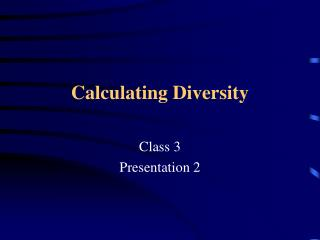DownloadDownload PresentationCalculating Diversity

# Calculating Diversity

Download Presentation## Calculating Diversity

- - - - - - - - - - - - - - - - - - - - - - - - - - - E N D - - - - - - - - - - - - - - - - - - - - - - - - - - -
##### Presentation Transcript

1. Calculating Diversity Class 3 Presentation 2

2. Outline • Lecture • Class room exercise to calculate diversity indices

3. Why quantify biodiversity? • Initially thought that more diversity = more stable ecosystem* • Now used to measure and track changes *MacArthur, R. 1955. Fluctuations of animal populations and a measure of community stability. Ecology 35:533-536

4. How do we measure biodiversity? • Use functional categories • Ecosystem, species, genetic • Use theoretical categories* • Alpha • Beta • Gamma * Whittaker, R.H. 1960. Vegetation of the Siskiyou Mountains, Oregon and California. Ecol. Mono. 30:279-338.

5. Alpha diversity • Diversity within a particular sample • E.g. the number of species surveyed

6. Beta Diversity • Changes in sample composition along an environmental gradient • E.g. composition of forest stands on the slope of a mountain

7. Gamma Diversity • Diversity due to differences in samples when all samples combined • E.g. diversity of a forest landscape

8. Describing Communities • Two methods • Describe physical attributes (e.g. age class, size class) • Describe number of species and their abundance

9. Biodiversity • Diversity of living things • Term often misused and overused • Current focus in conservation studies • Includes interest in genetic, species and ecosystem diversity • We will use species as our focus but concepts can be used for genetic and ecosystem diversity as well.

10. Species Richness • Number of species in a community • The simplest measure • Can count all spp only is few simple ecosystems • Does not consider number of individuals • Difficulties • When is it a specie? • Aphids • Clonal plants • Cannot count all species with limited time

11. Species Richness • How? • Identify organism groups of interest • Identify boundaries of community • Survey area for organisms of interest

12. Species diversity • Species richness not very informative • Each community has 5 spp & 50 individuals

13. Diversity indices • To get a better description of the community we need to get a measure of spprichness and evenness of their distribution • We usually use an index to represent several different measures • E.g. stock markets, air pollution, etc.

14. Diversity indices • Over 60 indices used in ecology • Indices used to measure proportional abundance • Two major forms: • Dominance indices (e.g. Simpson index) • Information indices (e.g. Shannon Weiner index)

15. Simpson Diversity Index (D) • Simpson’s index considered a dominance index because it weights towards the abundance of the most common species. • measures the probability two individuals randomly selected from a sample will belong to the same category • For example, the probability of two trees, picked at random from a tropical rainforest being of the same species would be relatively low , whereas in the boreal forest would be relatively high.

16. Simpson Diversity Index (D) Ds = (n1(n1 -1)/N(N-1)) Where: Ds = Bias corrected form for Simpson Index n1 = number of individuals of spp 1 N = Total number of spp in community In this form as diversity increases index value gets smaller

17. Simpson Diversity Index (D) • To make it easier to read the index is often read as: • Reciprocal i.e. 1/ Ds • Complimentary form: 1- Ds • Here as diversity increases Index value increases

18. Simpson Diversity Index (D) See Excel Show how index changes ((56*55)/(125*124))+ ((48*47)/(125*124)) + …………. ….((3*2)/125*124)) = 0.35509

19. Simpson Diversity Index (D) • Complimentary form = 1-D • = 1-0.35509 = 0.6449 • Reciprocal 1/D • 1/0.35509 = 2.816

20. Shannon-Weiner Index (H') • The index measures the uncertainty of a category in a particular set • It is a measure of evenness • For example, very low uncertainty the letter y is the next letter in this string: yyyyyyy (H' = 0)

21. Shannon-Weiner Index (H') • Assumptions: • All species represented • Sample randomized (equal probability of being selected in the sample) H' =- pilnpi pi= proportion of the ith species ln=natural logarithm

22. Shannon-Weiner Index (H')

23. Shannon-Weiner Index (H') • Index affected by both number of species and evenness of their population • Diversity increases as both increase • Diversity maximum when all species equally abundant

24. Evenness • Can use Shannon Weiner index to get a measure of evenness • First calculate Hmax • Evenness = H‘/ Hmax • Evenness will vary between 1 and 0

25. Evenness • In the last example • H‘= 1.1875 • Hmax = 1.609 • Therefore E = 1.1875/1.609 = 0.738 • The closer to 1 the more even the populations that form the community

26. Questions?